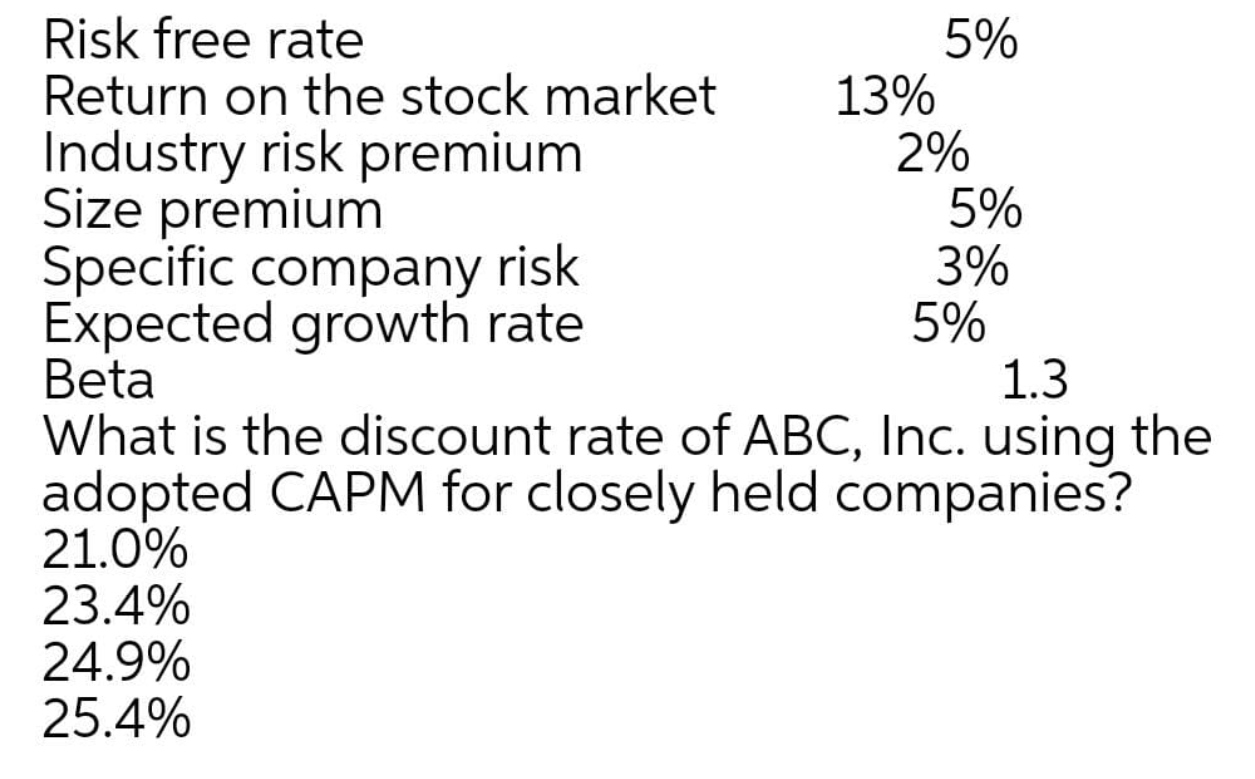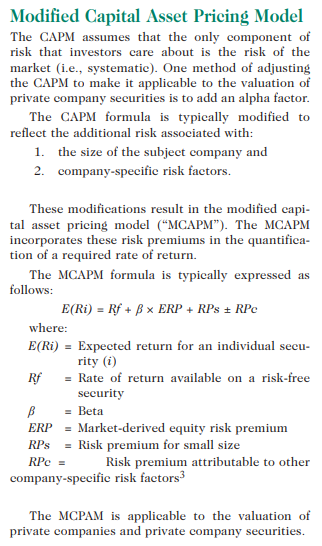# CMA Study Group

View Only

## Calculate the CAPM.#### Prajnya Shetty17 days ago#### Michael Henry16 days ago• #### 1.  Calculate the CAPM.

Posted 17 days ago
Hi All,

Can anyone answer the below question with explanation.------------------------------
Prajnya Shetty
GL Executive in XPO Logistics
India.
------------------------------

• #### 2.  RE: Calculate the CAPM.

Posted 16 days ago
Based on the below, I get 23.4% as the discount rate.
We utilize the CAPM formula and add an alpha to modify for company-specific risks.
Ignore the industry risk as it is represented as part of Beta already.

R(i) = R(f) + ß(R(m) - R(f)) + R(s) + R(c)
where R(i) = Company Rate and R(s) = Size premium and R(c) = company specific

R(i) = 5 + 1.3(13-5) + 5 + 3
= 23.4%------------------------------
Michael Henry
Controller
------------------------------

• #### 3.  RE: Calculate the CAPM.

Posted 15 days ago
Thank you Michael

------------------------------
Yasser Elsayed Omar
Director/Manager
------------------------------